Fractions (Exercise – 7.2)Ex 7.2 Question 1.

Draw number lines and locate the points on them.Divide the number line from 0 to 1 into four equal parts

C = 2 / 4 = 1 / 2

B = 1 / 4

D = 3 / 4 and

E = 4 / 4 = 1Divide the number line from 0 to 1 into eight equal parts

B = 1 / 8

C = 2 / 8

D = 3 / 8

H = 7 / 8From the given number line, we have

C = 2 / 5

D = 3 / 5

E = 4 / 5

I = 8 / 5

Ex 7.2 Question 2.

2. Express the following as mixed fractions:

(a) 20 / 3

(b) 11 / 5

(c) 17 / 7

(d) 28 / 5

(e) 19 / 6

(f) 35 / 9

Solution:3. Express the following as improper fractions:

(a)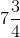(b)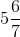(c)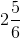(d)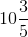(e)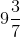(f)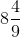Solution: